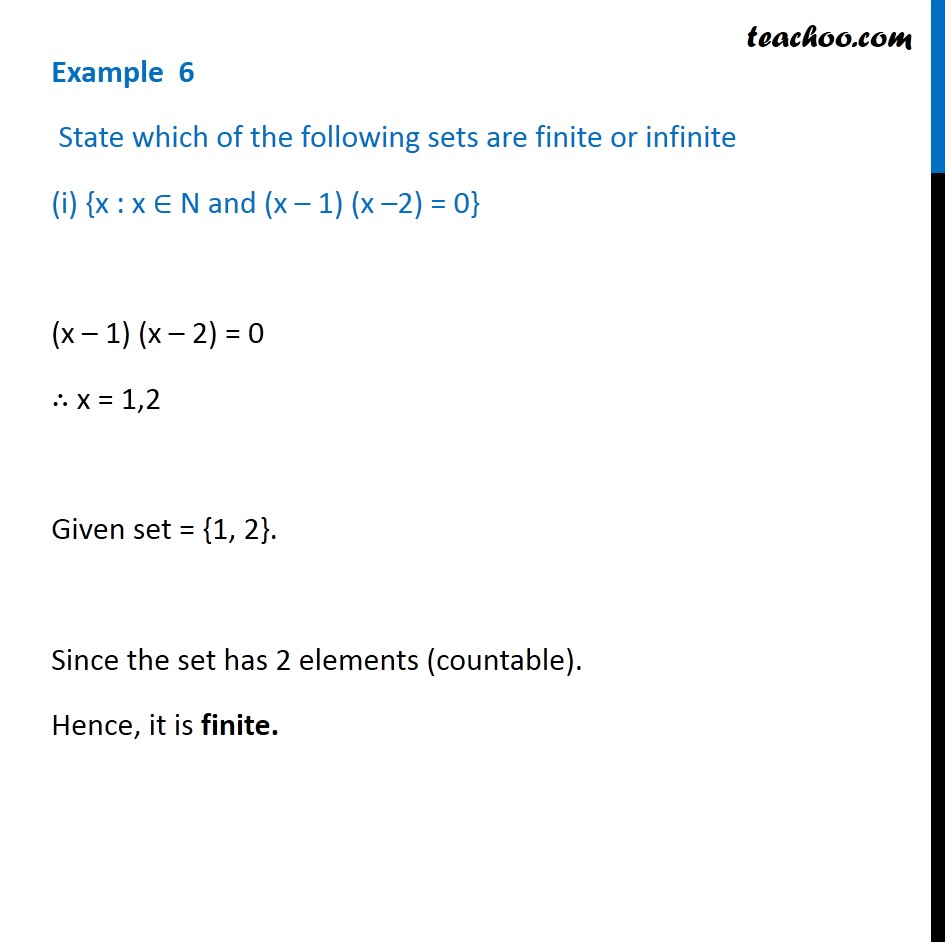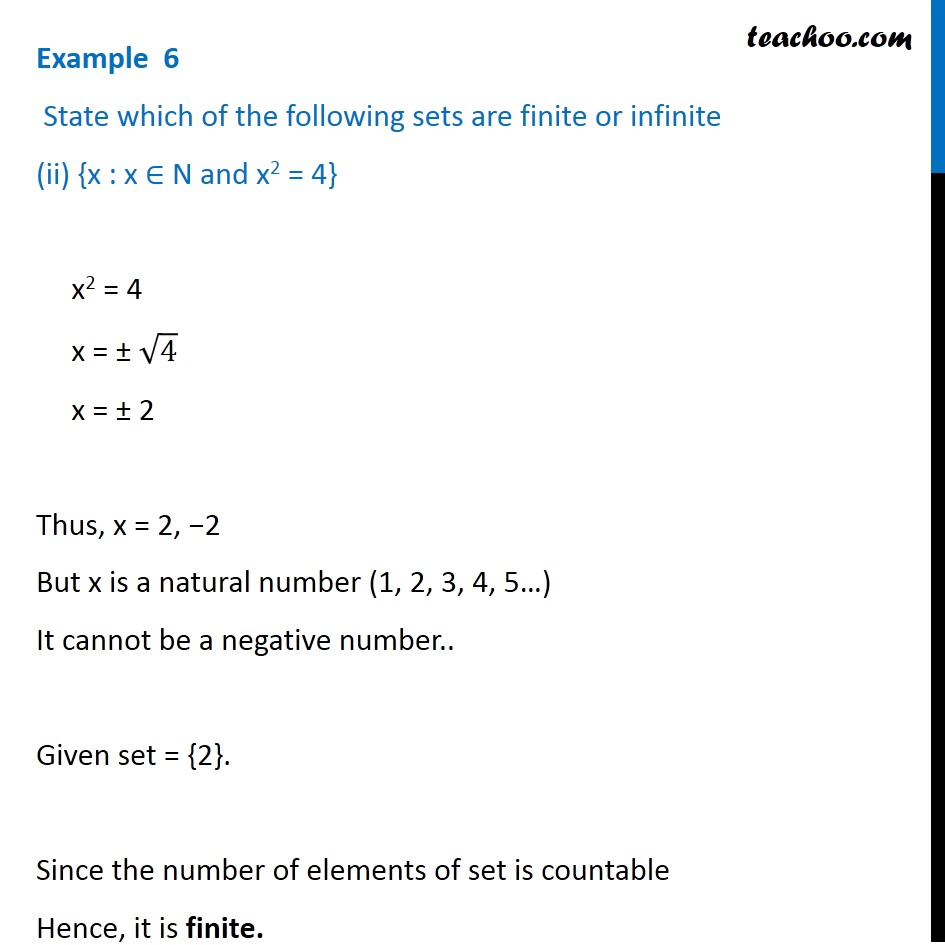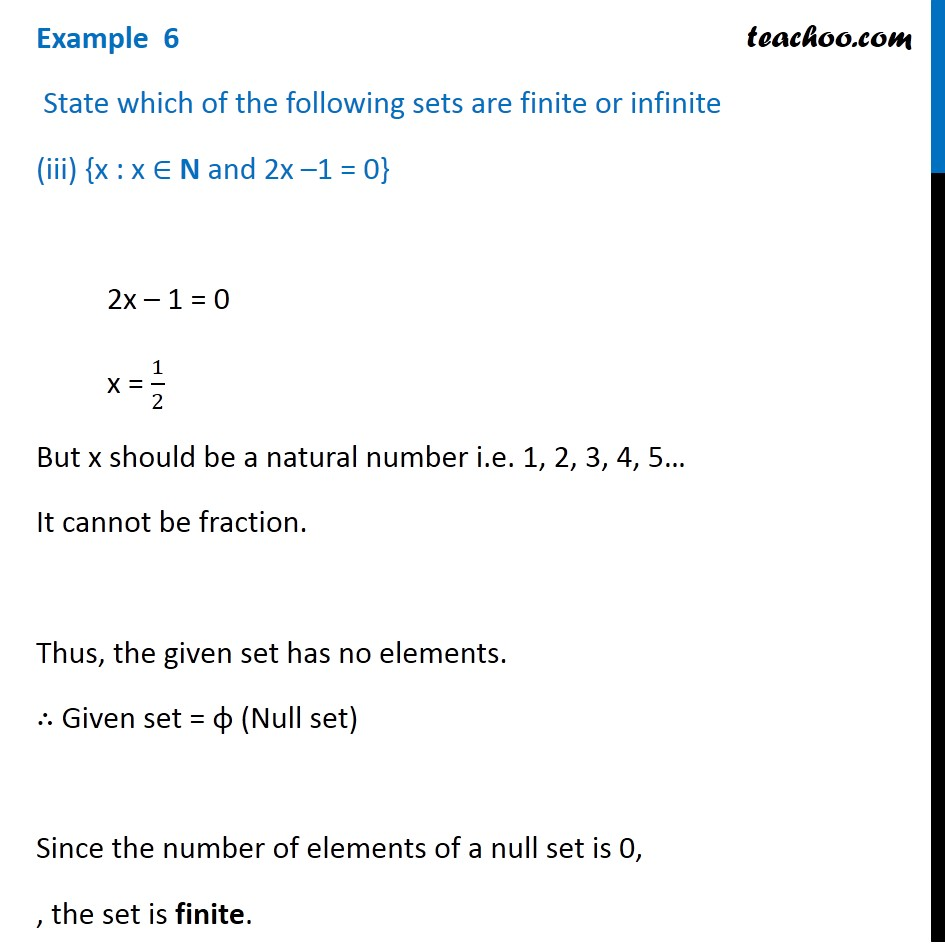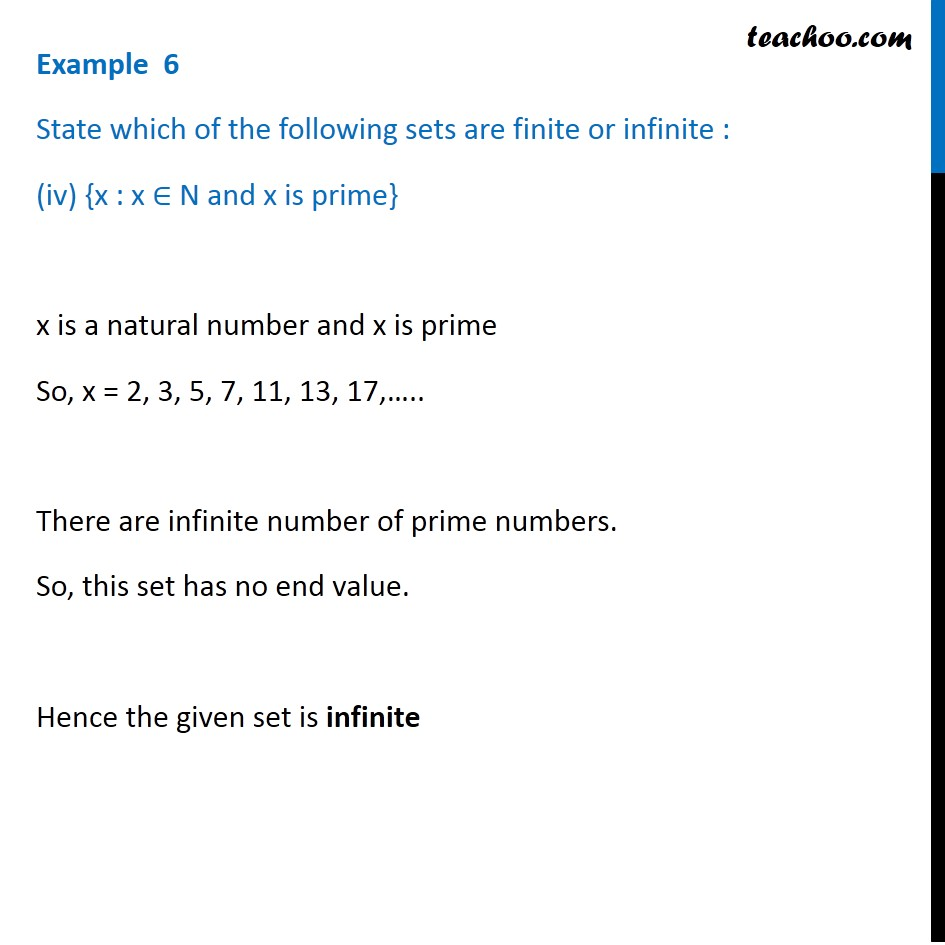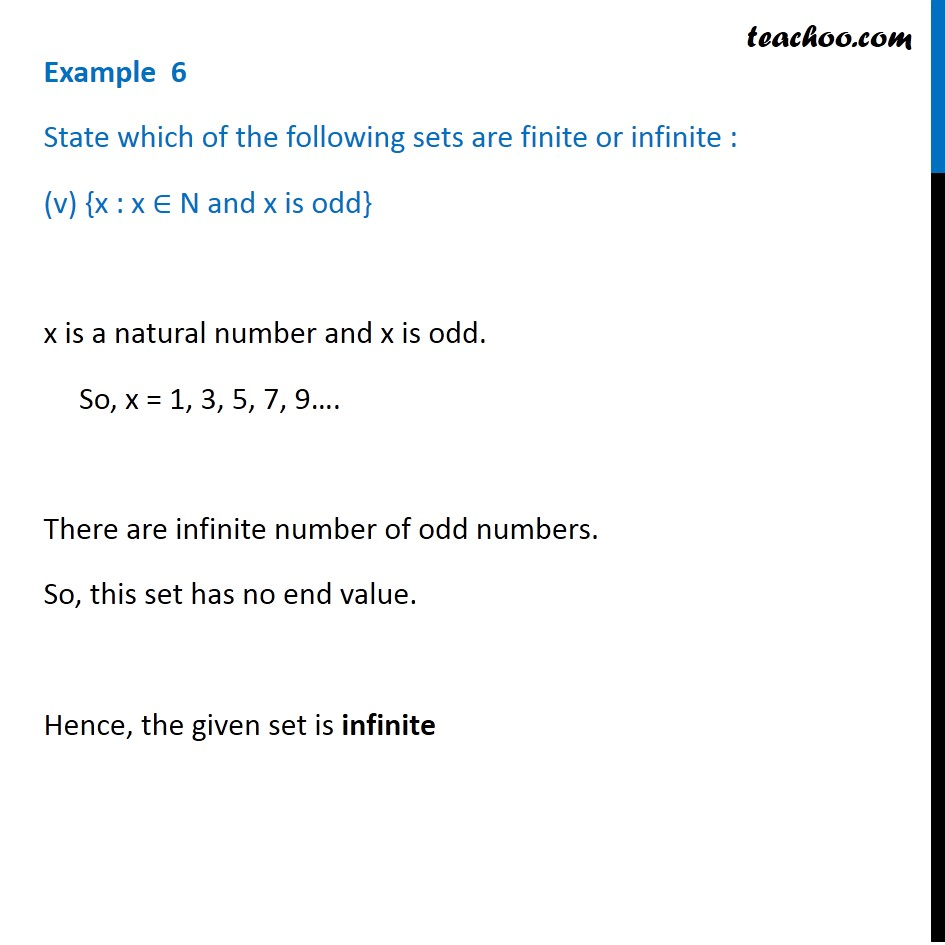1. Chapter 1 Class 11 Sets
2. Serial order wise
3. Examples

Transcript

Example 6 State which of the following sets are finite or infinite (i) {x : x ∈ N and (x – 1) (x –2) = 0} (x – 1) (x – 2) = 0 ∴ x = 1,2 Given set = {1, 2}. Since the set has 2 elements (countable). Hence, it is finite. Example 6 State which of the following sets are finite or infinite (ii) {x : x ∈ N and x2 = 4} x2 = 4 x = ± √4 x = ± 2 Thus, x = 2, −2 But x is a natural number (1, 2, 3, 4, 5…) It cannot be a negative number.. Given set = {2}. Since the number of elements of set is countable Hence, it is finite. . Example 6 State which of the following sets are finite or infinite (iii) {x : x ∈ N and 2x –1 = 0} 2x – 1 = 0 x = 1/2 But x should be a natural number i.e. 1, 2, 3, 4, 5… It cannot be fraction. Thus, the given set has no elements. ∴ Given set = φ (Null set) Since the number of elements of a null set is 0, , the set is finite. Example 6 State which of the following sets are finite or infinite : (iv) {x : x ∈ N and x is prime} x is a natural number and x is prime So, x = 2, 3, 5, 7, 11, 13, 17,….. There are infinite number of prime numbers. So, this set has no end value. Hence the given set is infinite Example 6 State which of the following sets are finite or infinite : (v) {x : x ∈ N and x is odd} x is a natural number and x is odd. So, x = 1, 3, 5, 7, 9…. There are infinite number of odd numbers. So, this set has no end value. Hence, the given set is infinite

Examples

Chapter 1 Class 11 Sets
Serial order wise